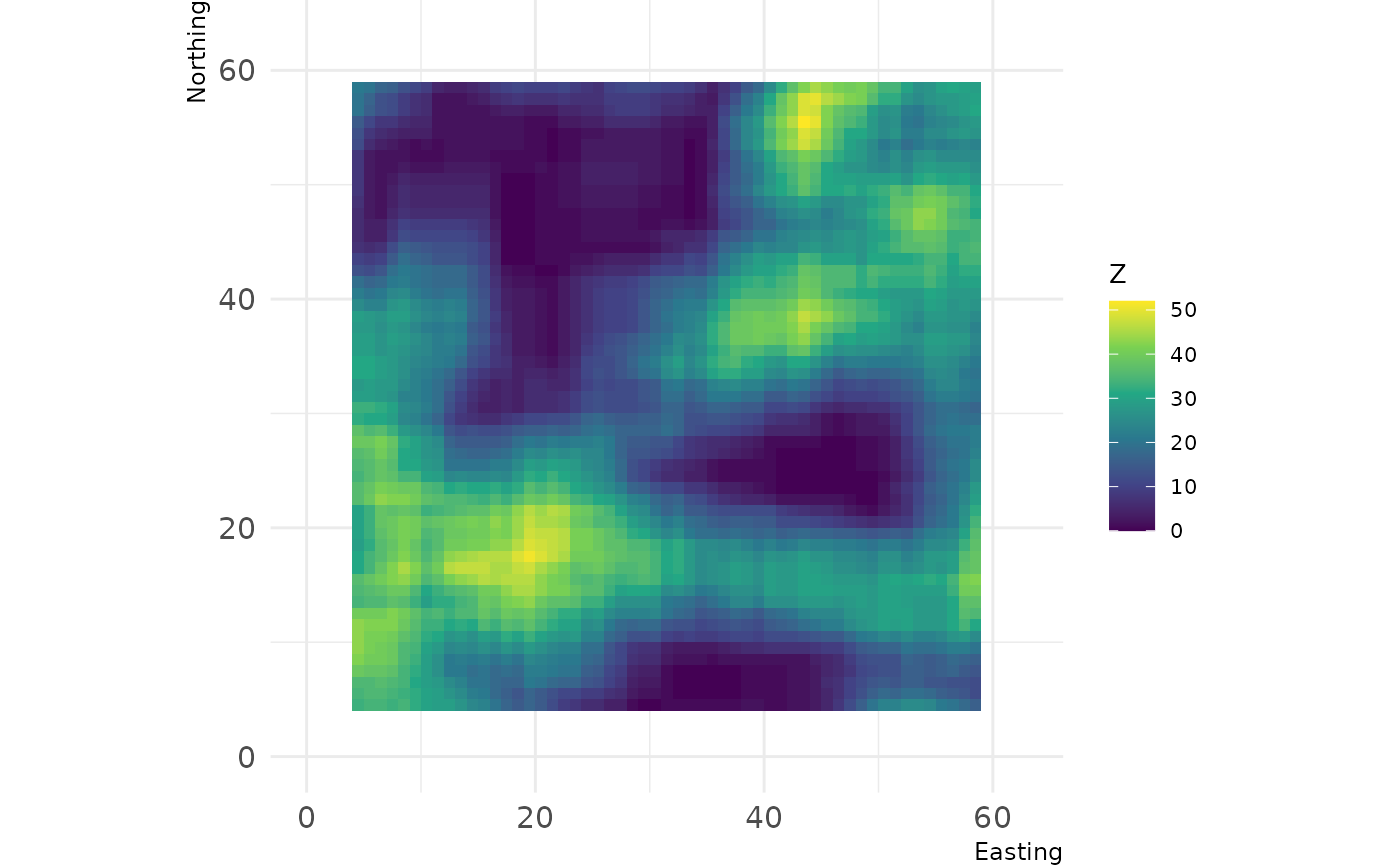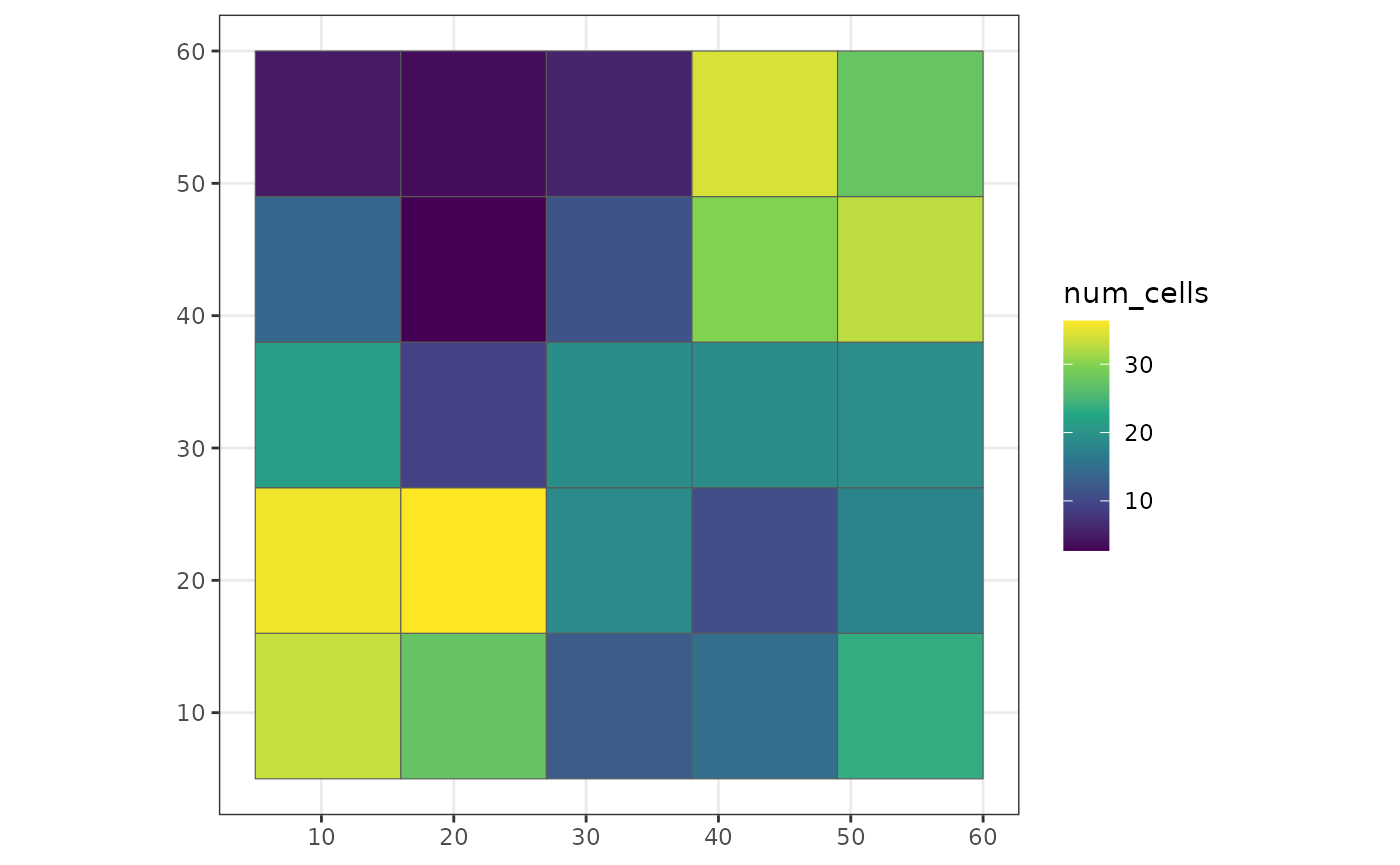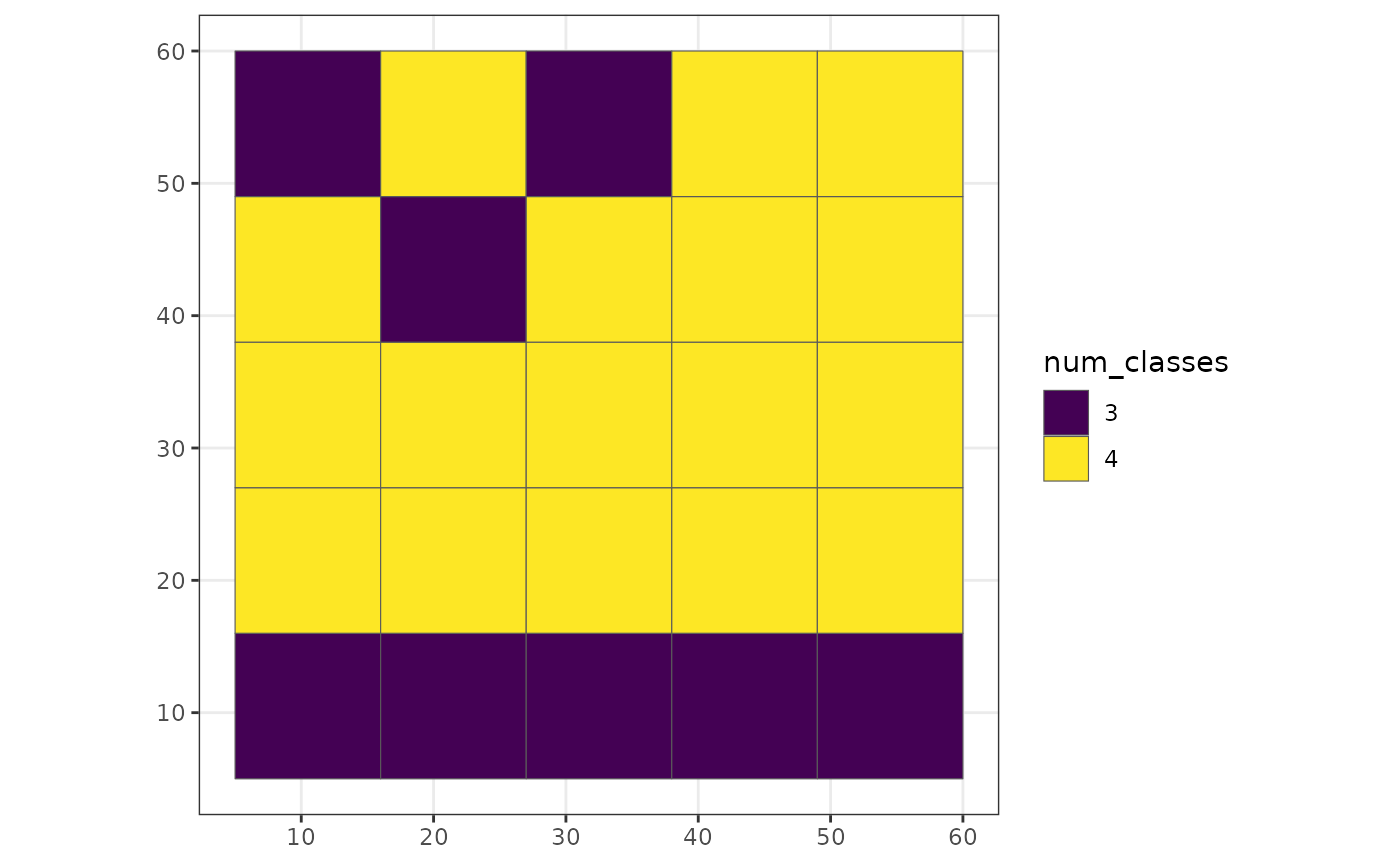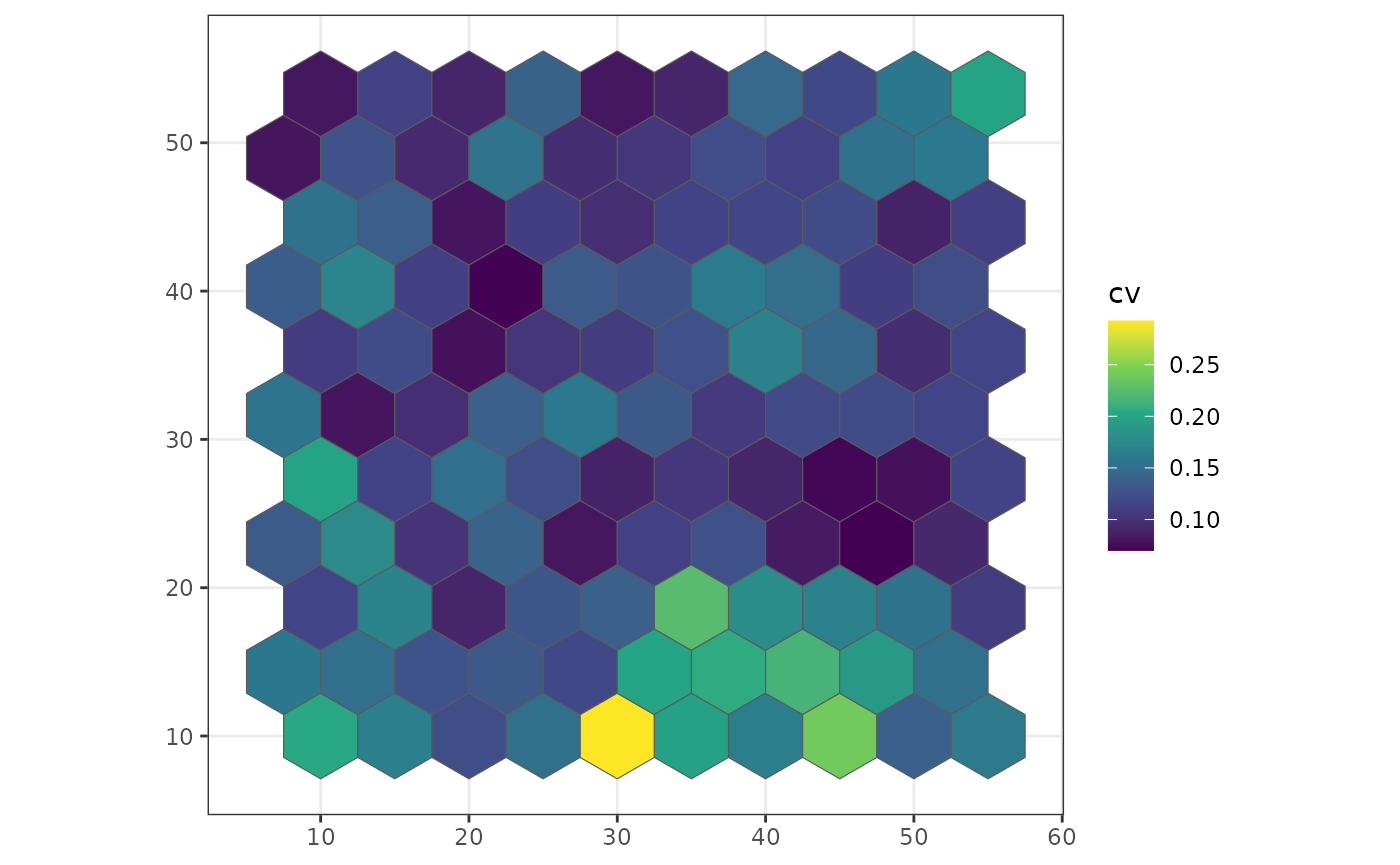Within the `winmove`, `winmove_agg` and `nomove_agg` functions, it is possible to use user-defined functions for both `win_fun` and `agg_fun` arguments.

WARNING User-defined functions can be slower within the `grainchanger` functions because they have not been optimised. This is likely to be of particular issue with large datasets.

## User-defined `win_fun` example

Any user-defined `win_fun` should follow the rules of the `fun` argument in `raster::focal`:

The function fun should take multiple numbers, and return a single number. For example mean, modal, min or max. It should also accept a na.rm argument (or ignore it, e.g. as one of the ‘dots’ arguments. For example, length will fail, but function(x, …){na.omit(length(x))} works.

In this example, we define a function which counts the number of cells of a given class within a moving window.

``````library(grainchanger)
library(landscapetools)

num_cells <- function(x, lc_class, ...) {
return(sum(x == lc_class))
}
d <- winmove(cat_ls, 4, "rectangle", num_cells, lc_class = 2)
show_landscape(d) ``````This can also be used within `winmove_agg`

``````library(ggplot2)
g_sf\$num_cells <- winmove_agg(g_sf, cat_ls, 4, "rectangle", num_cells, lc_class = 2)
#> Warning: aggregation assumes all cells are rectangular
#> • set `is_grid = FALSE` if coarse_dat is not a grid
#> Warning: Moving window extends beyond extent of `fine_dat`
#> You will get edge effects for the following cells of `coarse_dat`:
#> 1,2,3,4,5,6,7,8,9

ggplot(g_sf, aes(fill = num_cells)) +
scale_fill_viridis_c() +
geom_sf() +
theme_bw()``````## User-defined `agg_fun`

In this example, we define a function which calculates the number of land cover classes within each coarse grain cell.

``````num_classes <- function(x, ...) {
length(unique(x))
}

g_sf\$num_classes <- nomove_agg(g_sf, cat_ls, num_classes)
#> aggregation assumes all cells are rectangular
#> • set `is_grid = FALSE` if coarse_dat is not a grid

ggplot(g_sf, aes(fill = as.factor(num_classes))) +
scale_fill_viridis_d("num_classes") +
geom_sf() +
theme_bw()``````We can also define functions which work on continuous landscapes. For example, below we calculate the coefficient of variation for each coarse cell.

``````cv <- function(x) {
sd(x) / mean(x)
}

poly_sf\$cv <- nomove_agg(poly_sf, cont_ls, cv)
#> aggregation assumes all cells are rectangular
#> • set `is_grid = FALSE` if coarse_dat is not a grid

ggplot(poly_sf, aes(fill = cv)) +
scale_fill_viridis_c() +
geom_sf() +
theme_bw()``````